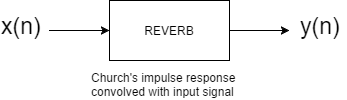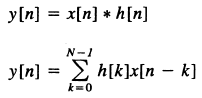This series is meant to outline some important digital signal processing topics as well as showcase one possible implementation of a real-time convolution reverb. We will discuss digital systems, FIR filtering, the FFT algorithm, and how to perform convolution in C++ efficiently and without significant input/output latency. But before that, it is worth describing convolution reverb itself.

To understand what convolution reverb is, picture yourself inside a cathedral. When you make a loud clap (an impulse), the room responds in its own specific, consistent way: the direct pressure waves from the clap smack against the nearest walls and shatter into many weaker pressure waves, creating a wash of reverberance.Though the input can be any sound (from a clap to a symphonic choir), the cathedral will always respond the same way, just like a black box. This consistent response to input signals from the church is known as an “impulse response” and can be captured digitally under controlled conditions by recording the space and introducing a single, isolated impulse across the audible frequency spectrum to capture how the space responds to all frequencies relevant to audio applications. (A sine wave sweep is most accurate but the white-noise impulse of a pistol will also work)

With digital signal processing, we can take this impulse response signal and convolve it with any input signal to make the signal sound like it was played in that cathedral.Convolution itself is just a mathematical operation like addition or multiplication, though used heavily in digital signal processing. For our purposes, convolution takes an input audio signal and filters its frequency spectrum with that of the impulse response. The mathematical expression for convolution is this:Convolution can be implemented in a number of ways, and we will discuss a solution with a combination of direct convolution and fast convolution, accounting for the performance drawbacks of both. In short, direct convolution directly processes an input signal with an FIR filter whose coefficients are all of the sample points of the impulse response. (Each input sample is multiplied by EVERY sample in the impulse response.) Can get very inefficient quickly, especially for longer impulse responses. Fast convolution takes advantage of the fact that convolution in the time domain is multiplication in the frequency domain; it takes the fast Fourier transform of the input signal, multiplies it with the frequency spectrum of the impulse response, and takes the inverse FFT to return the signal to the time domain for output. The drawback here is that the FFT requires a specified threshold of input samples before it can begin processing, and thus causes significant latency. We will see that the convolution processing can be partitioned in such a way that the FIR convolution is performed until the FFT gets its required input threshold, solving both latency and inefficiency issues.